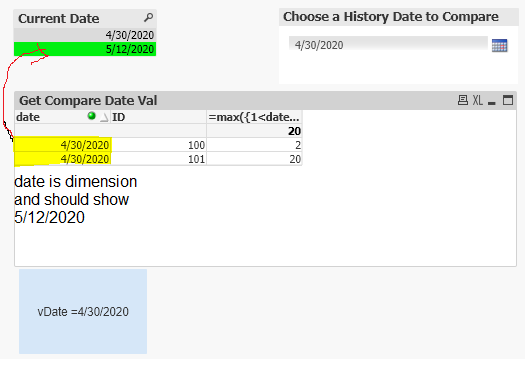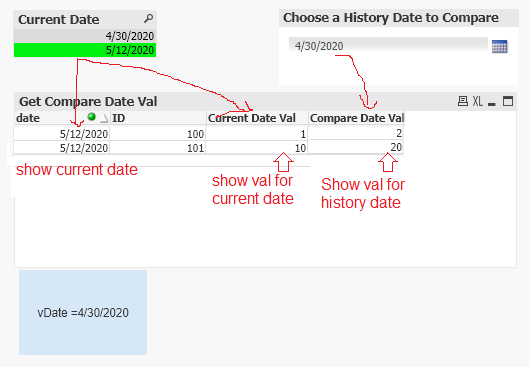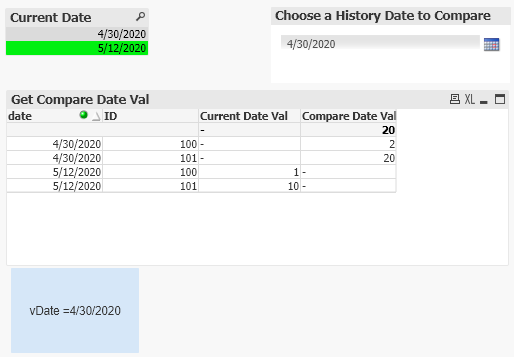# New to QlikView

Discussion board where members can get started with QlikView.

Announcements
Modernize Your QlikView Deployment webinar, Nov. 3rd. REGISTER
cancel
Showing results for
Did you mean:
HighlightedCreator

## dimension is changed by set analysis ??

Hi experts,

I got a strange problem in a very simple data set. qvw is attached.

My data set is very simple, just like below:

'data':
date,ID,val
'5/12/2020', 100, 1
'4/30/2020', 100, 2
'5/12/2020', 101, 10
'4/30/2020', 101, 20
]

in dashboard, I put a list box for "date" field so user can select a date. I put a calendar which is connected to a variable vDate. Then I have a table, dimension is "date" and "ID", express is like below:

=max({1<date={'\$(=Date(\$(vDate)))'}>} val)

Note I use "1" and date in the set analysis. basically what I want is , when date is selected as 5/12/2020, and calendar is set to be 4/30/2020, I want the table to show the val of 4/30. It shows the correct val but why the date column is 4/30? It should be 5/12 because I'm selecting 5/12 in the list box, right?the qvw file is attached.

Thanks,

Michael

Labels (1)
• ### Set Analysis

2 Solutions

Accepted Solutions
HighlightedMVP

Try this

``=Aggr(Above(Max({1}val)), ID, (date, (numeric)))``
HighlightedMVP

Try this

``=Aggr(Above(Max({1<date = {"\$(=Date(\$(vDate)))", "\$(=Date(Max(date)))"}>}val)), ID, (date, (numeric)))``
6 Replies
HighlightedMVP

May be try this

``=Above(Max({1<date={'\$(=Date(\$(vDate)))'}>} val))``
HighlightedCreator

hi,

this seems to be not working. What I want is actually something like below,but my expression gives me this. I don't understand why 4/30 rows are in the table even though I have selected the date 5/12 in list box.HighlightedMVP

Try this

``=Aggr(Above(Max({1}val)), ID, (date, (numeric)))``
HighlightedCreator

Hi,

thank you for the solution. but i just found this only works when I have two dates, what if I have more dates and I want user to choose different Compare Date from the calendar and the table should be updated automatically?

a new qvw is attached with more data, I tried modifying the expression, but can't get it work..

Thanks,

Michael

HighlightedMVP

Try this

``=Aggr(Above(Max({1<date = {"\$(=Date(\$(vDate)))", "\$(=Date(Max(date)))"}>}val)), ID, (date, (numeric)))``
HighlightedCreator

Thank you!